# College Chemistry : Calculating Solubility

## Example Questions

### Example Question #1 : Calculating Solubility

What are the products of the following reaction?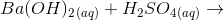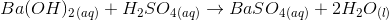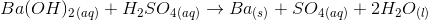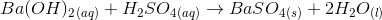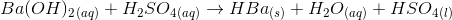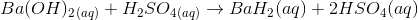Explanation:

The correct answer isFirst of all, we know this is an acid-base reaction.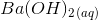is the reactant base and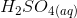is the reactant acid.  We know that these acid-base reactions create a water and a salt.  Also, we know that sulfates are soluble, except for those of calcium, strontium, and barium.  As a result,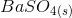is a precipitate in the reaction and water is produced.

### Example Question #2 : Calculating Solubility

Which of the following are soluble?

Chlorides

Hydroxides

Carbonates

Sulfides

Phosphates

Chlorides

Explanation:

Most salts containing halogens are soluble.  Carbonates, phosphates, sulfides, oxides, and hydroxides are insoluble except for cations containing alkaline earth metals and hydroxides of calcium, strontium, and barium, which are slightly soluble.

### Example Question #3 : Calculating Solubility

Calculate the molar solubility of calcium hydroxide. Calcium hydroxide has a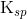value of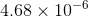.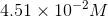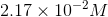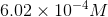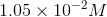Explanation:

Start by writing the equation for the dissolution of calcium hydroxide.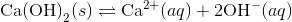Next, set up the following table to show the equilibrium concentrations of the ions:

 [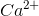] [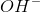] Initial 0.00 0.00 Change +S +2S Equilibrium S 2S

Now substitute in the values of the concentrations of the ions into the expression to find.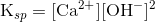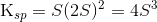Now, plug in the givenvalue and solve for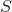, the molar solubility.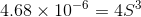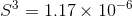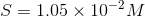### Example Question #4 : Calculating Solubility

Consider an aqueous solution that is saturated with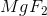. If the concentration of fluoride in this solution were cut in half, by how much would the magnesium concentration need to be changed in order for the solution to remain saturated?

The magnesium concentration would need to be decreased by a factor ofThe magnesium concentration would need to be increased by a factor ofThe magnesium concentration would need to be increased by a factor ofThe magnesium concentration would need to be decreased by a factor ofThe magnesium concentration would need to be increased by a factor ofExplanation:

For this question, we're told that an aqueous solution of magnesium fluoride is saturated. We're then told that the fluoride concentration in the solution is reduced by a factor of two, and we're asked to find how the magnesium concentration would need to change in order to keep the solution saturated.

First, it's important to recall what saturation means. Some substances cannot dissolve in water, whereas others can dissolve readily. In other words, different substances will dissolve to differing degrees in water. The dissolution of a compound in a solvent such as water can be represented by an equilibrium expression. When there is a relatively small amount of solute added, the solution is said to be unsaturated. This means that all of the added solute will dissolve. As more and more solute is added to the water, there will eventually reach a point at which so much solute is present that it can no longer dissolve. When this happens, any additional solute will not dissolve and will instead form a precipitate in the solution. This condition is referred to as supersaturated. Saturation is the "sweet spot" so to speak; it is the point in between unsaturated and supersaturated where the maximum amount of solute has been added to the solution where all of the solute can be in the dissolved form. In other words, saturation refers to the maximum concentration of added solute where there is NO precipitation.

In order to set up an equilibrium expression, we can first write out the reaction in which magnesium fluoride dissolves.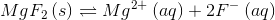Knowing the reaction, we can now write an equilibrium expression. Remember that pure solids and liquids don't appear in equilibrium expressions! Thus, this expression will only contain the products of the above reaction.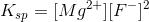In the above expression,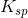refers to the solubility product constant, which is just a quantitative way of expressing the degree to which a given compound can dissolve within a given solvent.

Since the equilibrium expression tells us the concentrations necessary to have a saturated solution, we can determine how a change in fluoride concentration would affect the equilibrium. Then, we can determine what changes to magnesium are needed.

If the fluoride concentration were to be cut in half, thevalue would be decreased by a factor of. This means that in order to maintain thevalue, we would have to increase the magnesium concentration by a factor ofin order to compensate for the loss of fluoride.